Balance Beam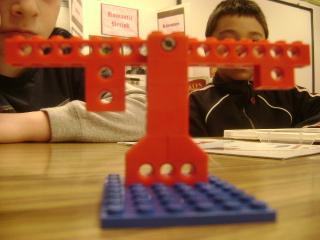April 18, 2011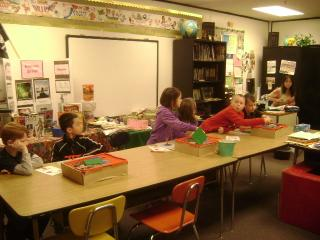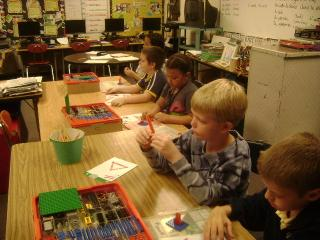First and second graders built the balance beam in the green B instructions for the simple motorized machine sets.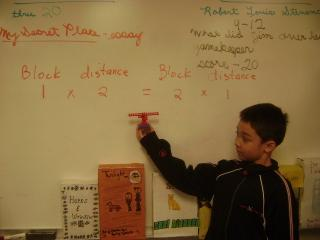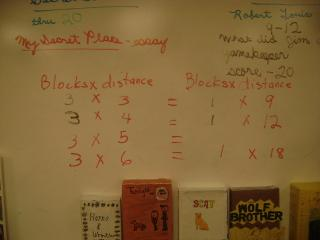Then they figured out many combinations of blocks and positions that balanced.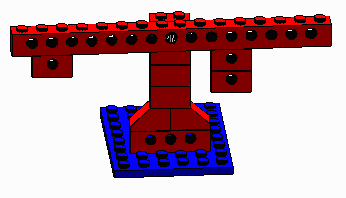1 X 6 = 2 X 3
Number of blocks times the distance from the middle on one side
equals
the number of blocks times the distance from the middle on the other side.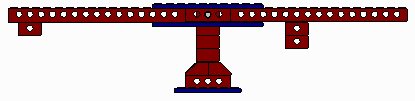1 X 16 = 2 X 8
To get larger numbers they used the idea from the Green A instructions for making longer beam
by sandwiching them between two plates.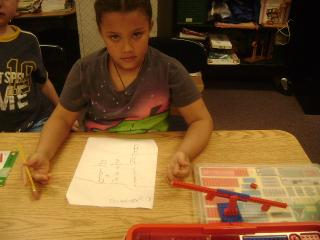6 + 6 + 6
is 9 + 9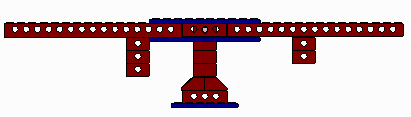3 X 6 = 2 X 9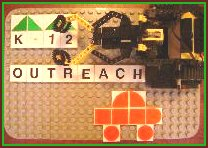of Linda Hamilton hamilton@marshall.edu With support from